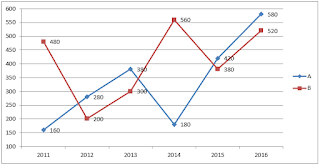# gkrecall

We brings you daily Current affairs, daily Current Affairs Quiz, weekly Current Affairs, weekly Current Affairs Quiz to enhance your preparation for upcoming exams. We also provide articles related to quant, reasoning, English, ssc etc .

## QUANT PRACTICE SET 8 FOR RRB PO & CLERK PRELIM 2020

RRB Clerk and RRB PO Prelims exam are going to be held in the upcoming months. We have already provided you with the PDFs of many topics of Quantitative Aptitude like Simplification/Approximation, Number Series,datainterpretation.important Arithmetic questions to prepare for RRB Clerk and PO Pre exam. Practicing these questions will help you to know about the level of the questions. To increase your speed and accuracy, enhance your calculations.

### START QUIZ

Q1. 12, 12, 18, 36, 90, 270, ?
853
820
945
980
720

Q2. 15, 30, ?, 40, 8, 48
10
12
18
20
7.5

Q3. 879, 536, ?, 195, 131, 104
250
296
315
385
320

Q4. 588, 563, 540, 519, ?, 483, 468
500
496
494
490
504

Q5. 33, 39, 57, 87, 129, ?
183
177
189
199
181

Directions (6-10): Refer the graph and answer the given questions.

The following line graph shows the number of votes polled in two constituencies A and B of a city during six years.Q6. What is the difference between number of votes polled in constituency A and B together in 2013 and number of votes polled in both constituencies together in 2011.
50
30
60
40
20

Q7. Find ratio of votes polled in constituency A in 2013 and 2014 together and votes polled in constituency B in 2015 and 2016 together.
19 : 21
28 : 45
9 : 26
45 : 28
28 : 29
Solution:
Votes polled in constituency A in 2013 and 2014 together = 380 + 180 = 560
Votes polled in constituency B in 2015 and 2016 together = 380 + 520 = 900
Q8. Number of votes polled in constituency A and B together in 2015 is what percent less or more than the number of votes polled in constituency B in 2015 and 2016 together.

Q9. If in 2012, 10% of total votes polled in constituency A are invalid and 5% of votes of constituency B in 2013 are invalid then find the average of valid votes of both constituencies.
268.5
267.5
283.5
272.5
270.5

Q10. Find average number of votes polled in constituency A in all the given years.
343.33
333.33
233.33
330.33
353.33

Directions (11-15):What approximate value will come in place of (?) in the following questions ?

Q11.525
418
460
432
455

97
58
81
72
61

Q13. 16.02² + 144+ 23.96 + ? = 783.867
316
262
258
360
344

1089
841
1369
289
529

17470
17496
18496
18086
18156

SOLUTIONS:

1.
2.
3.
4.
5.
6.
Number of votes polled in constituency A and B in 2013 = 300 + 380 = 680
Number of votes polled in both constituencies in 2011 = 160 + 480 = 640
Required difference = 680 – 640 = 40

7.
Votes polled in constituency A in 2013 and 2014 together = 380 + 180 = 560
Votes polled in constituency B in 2015 and 2016 together = 380 + 520 = 900
8.
Number of total votes in both constituencies in 2015 = 420 + 380 = 800